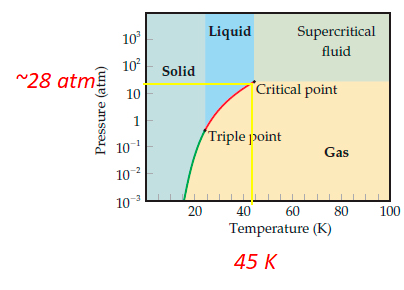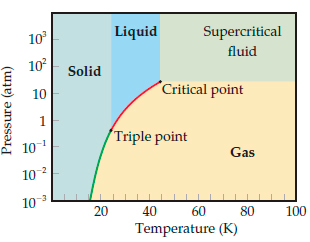# Problem: Use the phase diagram of neon to answer the following questions.What can you say about the strength of the intermolecular forces in neon and argon based on the critical points of Ne and Ar? (critical temperature (Ar) = 150.9  K, critical pressure (Ar) = 48.0  atm)

###### FREE Expert Solution

Recall that a phase diagram shows the transition of matter between solid, liquid, and gas phases as temperature and pressure changes.

The critical point is the pressure and temperature at which the liquid and gas phase are indistinguishable (gas and liquid phase ends)

For Neon, critical point: Tc = ~45 K, Pc = ~28 atm###### Problem Details

Use the phase diagram of neon to answer the following questions.What can you say about the strength of the intermolecular forces in neon and argon based on the critical points of Ne and Ar? (critical temperature (Ar) = 150.9  K, critical pressure (Ar) = 48.0  atm)